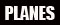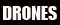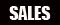# Control Tower - January 1999

### Jim Bourke presents Part 5 of "Understanding Electric Power Systems"

Understanding Electric Power Systems - Part 5

This month I present part 5 of my series on electric power systems.  Please refer to my September 1998 column if you are looking to catch up on missed information.

Recap

In the December, 1998 edition of this series I presented the concept of electrical resistance and explained its effect on motor RPM.

Here are the answers to last month's Q&A:

 Q&A Suppose I have two motors with the same Kv and Rm.  Both of them are being run on 10 cells.  The first motor is spinning its propeller at 10,000 RPM.  The second motor is spinning its propeller at 9,500 RPM.  Which motor is spinning the larger propeller? The second motor is being used to spin a larger propeller.  The larger propeller provides more of a load, which causes more current to be drawn, which causes the RPM to drop.   Which motor will spin faster on 10 cells at 20 amps: a motor with a Kv of 1000 and an Rm of .050 Ohms or a motor with a Kv of 950 and an Rm of .010 Ohms? Lets examine both cases: Motor A has a Kv of 1000 and an Rm of .050 Ohms.  The RPM will be: RPM = Kv * (V - Vloss) RPM = Kv * (V - I * Rm) RPM = 1000 * (10 - 20 * .050) RPM = 1000 * (10 - 1) RPM = 1000 * 9 RPM = 9000 Motor B has a Kv of 950 and an Rm of .010 Ohms.  The RPM will be: RPM = Kv * (V - I * Rm) RPM = 950 * (10 - 20 * .010) RPM = 950 * (10 - 0.2) RPM = 950 * 9.8 RPM = 9310 Surprisingly, Motor B will produce more RPM!  This example demonstrates the importance of armature resistance in predicting motor performance.   Joe is walking out to retrieve his Super-Thermal-2000 R/C electric sailplane at his local field when he accidentally bumps the throttle switch.  The propeller is unable to move.  He is using an 8 cell pack and a speed 600 motor with an Rm of .145 Ohms.   How much current will the motor attempt to draw in this stalled condition? We compute the answer using the formula for stall current shown in last month's column: Istall = Vin / Rm Istall = 8 / .145 Ohms Istall = 55 Amps

Losses and Limitations, continued

Last month I mentioned that there are 4 main losses and limitations we must consider when attempting to predict motor performance.  They are:

1. Armature Resistance
2. No load current
3. RPM limit
4. Torque limit

Last month's column described the effects of Armature Resistance.  This month I would like to discuss the remaining three parameters of our simplified motor model.  Before I can do so, I must explain some basic physics.

Power, Work, and Torque

If you were tasked to lift a heavy box up a flight of stairs to a platform, you would have to accomplish work.  To measure that work, we could take the weight of the box and multiply it by the height of the platform.  For instance, if you lifted a 10 lb box up 100 feet of stairs, we could say that you did 1000 foot lbs of work.  We could also say you did 1000 foot lbs of work if you lifted a 20 lb box up 50 feet of stairs, or if you lifted 5 boxes, each weighing 10 lbs, up 20 feet of stairs.  All of these activities involve the same amount of energy expenditure and the same amount of work.

1000 foot pounds of work will:

• lift a 10 pound box up 100 feet of stairs.
• lift a 20 pound box up 50 feet of stairs.
• lift a 10 pound box up 20 feet of stairs 5 times.

If the amount of time at your disposal was essentially unlimited, you could accomplish all of the above work without becoming tired.  But there is a limit to how much work you can do within a specific time period.  We can all agree, for example, that it is much harder to run a 26 mile marathon in a few hours than it is in a few days even though the same amount of work is accomplished.

Power is a measure of a machine's ability to do work over time.  100 foot pounds per second of power would accomplish 100 foot pounds of work every second.  Thats enough power to lift 1 pound up 100 feet of stairs every second or 100 pounds up 1 foot of stairs every second.  As long as we did 100 foot pounds of work every second we would be absorbing 100 foot pounds per second of power.

100 foot pounds per second of power will:

• lift a 10 pound box up 10 feet of stairs in 1 second
• lift a 100 pound box up 100 feet of stairs in 100 seconds
• lift a 5 pound box up 5 feet of stairs 4 times a second

In the US, we tend to think in terms of horsepower, so it might help to know that a single horsepower is equivalent to 550 foot lbs of work every second.

1 horsepower = 550 foot lbs/sec

In other words, if I had a machine that could produce one horsepower, it could lift 5.5 lbs up a 100 foot staircase every second.  That is much, much faster than a human being is able to work.

In electric flight we rarely ever deal with horsepower, except when we are comparing our power systems to something in the internal combustion world.  Instead, we tend to visualize power using watts.  One horsepower is roughly equal to 746 watts.

1 horsepower = 746 watts

A watt is a watt

At this point in this series on electrical power, we have used the word "watt" to mean both electrical power and mechanical power.  It turns out that a watt is a unit of power that can express ANY kind of power, not just the stuff coming out of our batteries.  I can take a watt of electrical power and, in a perfect world, convert that watt to exactly one watt of mechanical power.  In fact, that is exactly the kind of endeavor our motors are designed to do.  They aren't anything more than a power converter.

However, the power coming out of our motors is not automatically able to lift boxes up a flight of stairs.  It must be further converted to accomplish that task.  That is because the power coming out of our motors is rotational power.  It is power expressed in terms of RPM and Torque.

• Watts = volts x amps
• Watts = work / time
• Watts = torque x RPM
• Watts is always some kind of work over time.
• Watts is watts!

When it comes to electricity, watts is the ability to do electrical work over time ("amps" contains the time component).

When it comes to rotational power, watts is the ability to do rotational work over time ("RPM" contains the time component).   RPM is a pretty easy concept to understand.  It is the revolutions per minute that the shaft is turning.  Torque, however, is a little trickier.  To put it simply, torque is the "oomph" with which the motor shaft turns.  As we have learned in this series, it is much harder to spin a large propeller at 10,000 RPM than it is to turn a small propeller at 10,000 RPM.  The larger propeller requires more torque.

How much torque does my motor produce?

Just as the amount of RPM produced by a motor is based on the amount of voltage applied, the amount of torque produced by a motor is based on the amount of current applied.  In fact, there is a motor constant to express torque as a function of current.  It is called Kt and is expressed in units of inch-ounces per amp (at least thats how we express it in the US, where this column is being written - you might be used to thinking of torque in terms of newton-meters if you use the metric system).

Lets take a look at the constants of some real world motors and see what we can learn about the torque constant, Kt:

 Table 5-1.   Aveox Motors and their constants. Motor Kv Kt Kv * Kt Aveox 1406/2Y 3000 .451 1352 Aveox 1406/3Y 2000 .676 1352 Aveox 1406/4Y 1500 .901 1352 Aveox 1409/3Y 1333 1.014 1352 Aveox 1409/4Y 1000 1.352 1352

Table 5-1 shows a small sample of Aveox motors and the Kv and Kt constants published on their web site at www.aveox.com.   Aveox sometimes gets a little grief from their customers over the naming convention of their motors, but in reality they have an excellent way to describe their motors and they do a great job of listing all the data we need to know precisely how the motor will perform.  The trick is to understand the motor constants, which you are on your way to doing.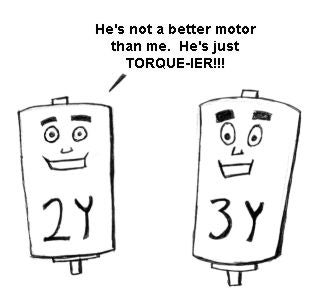Note that the 5 motors listed all have different Kv values.  Note that the Kv goes from 3000, in the case of the 1406/2Y motor, down to 1000, in the case of the 1409/4Y motor.  The Kv decreases as we move down the table.  Note that at the same time, the Kt increases as we move down the table, from .451 inch ounces per amp in the case of the 1406/2Y all the way up to 1.352 inch ounces per amp in the case of the 1409/4Y.

The fourth column really tells the whole story.  It turns out that Kt and Kv are interrelated in such a way that it is impossible to increase one without decreasing the other proportionately.  The product of Kt and Kv is a constant for every motor.

Kt x Kv = 1352

So the amount of torque produced per amp is determined by the Kv of the motor.   The higher the Kv, the lower the amount of torque produced per amp.  The lower the Kv, the higher the amount of torque produced per amp.

• big Kv = low torque per amp
• little Kv = high torque per amp

It is impossible to produce a motor that provides a high Kv and a high amount of torque per amp.  If the Kv is high the motor will always have to draw lots of current to produce lots of torque.  If the Kv is very low then the motor will need lots of cells to produce lots of torque.

None of this is news

If you are getting confused by all this talk of torque then go back and re-read the first few columns in this series.  You will notice that all of this torque stuff is just a way of labelling what you already have learned about power.  You already knew, for instance, that a high-Kv motor would draw a lot of current to swing a big propeller.   The only difference is that you now know the reason is because a high-Kv motor has a low torque constant and needs a lot of current to produce a lot of torque.

Torque losses

Last month I showed how armature resistance caused an RPM loss.  It turns out that there is an analogous torque loss within the motor as well.  This loss is called the "no-load current" and is abbreviated as Io.

In the Ideal World, we can imagine an Ideal Motor which produces precisely as much torque as its Kt value dictates.  In reality, the amount of torque produced is always a little less than this ideal torque, just as the actual RPM is a little less than the ideal RPM.  There is a constant, called Io or "no load current", which works against the input torque.

So, instead of calculating torque using:

Torque = Kt * Iin

We calculate it using:

Torque = Kt * (Iin - Io)

The Io value dictates a torque loss that decreases motor efficiency, causing a real world motor to draw more current than an ideal motor would given the same propeller.   Any input current below the Io value results in a negative net torque coming out of the motor.  This is an important loss that must be considered when we attempt to predict the performance of an electric power system.

Limitations

Anyone who has ever "smoked" a motor can tell you that there is a certain point at which a motor cannot handle any more input power.  In reality, a motor is not limited by power, per se, but by RPM and torque.

The RPM limit of a motor is based on the ability of the armature to hold together under the centripetal forces caused by its rotation.  There is a limit to how much RPM a motor can handle.  A high quality motor might be able to survive 40, 50, or even 60,000 RPM.  Most electric flight motors are rated somewhere around 30,000 RPM.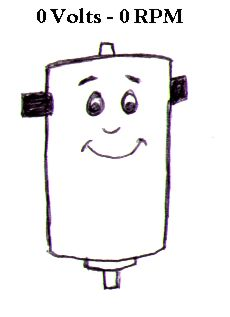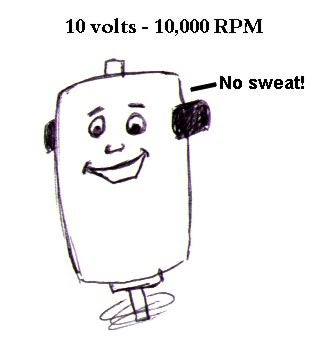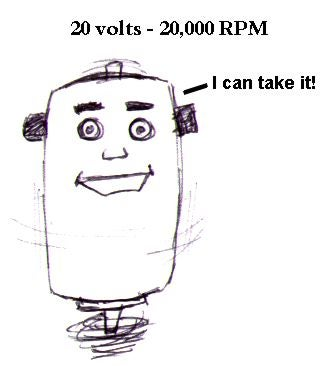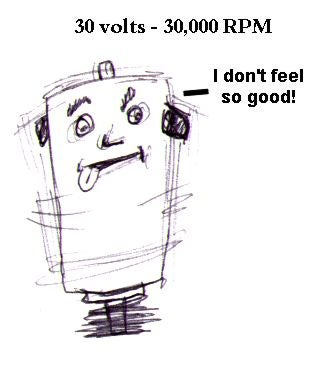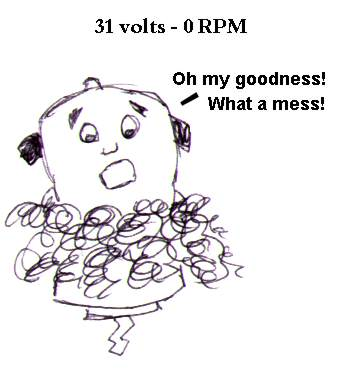Some modelers choose to operate their motors near the RPM limit, because a motor is typically more efficient at high RPMs.

There is a second limitation we will consider in our motor model called the torque limit.  The torque limit of a motor quantifies the the motor's ability to withstand heat.   One must remember that the production of torque necessarily requires current to be drawn through the motor.  Due to resistance, current causes heat to build up within the motor.  Over time this heat can grow to the point where it causes damage.   Most manufacturers publish a torque limit that will allow the motor to survive a typical R/C flight of a few minutes.  It is usually possible to go over this limit for short periods, which is how many competition flyers are able to push their motors into the 70 amp range.  Some manufacturers publish two torque limits: one for short term use and one that guarantees safe operation indefinitely.

Beware of manufacturers that do not publish the RPM and torque limits of their motors.   This is important information that you should demand as a consumer before purchasing any motor.  It is every bit as important as the armature resistance or Kv value.

Output Power

Now that we know all about losses and limitations, we can take a look at how much power our motors can produce given the input power provided by our batteries.

In an Ideal Motor, we had only one equation for power:

Watts = Volts x Amps

In the real world we must consider input power and output power separately.  The input power is the same as the Ideal case: it is the voltage and current out of the battery.  The output power, however, must consider the losses within the motor.

Output Watts = (Volts - Voltage losses) x (Amps - Amperage losses)
Power Out = (V - Vloss) * (Iin - Io)
Power Out = (V - Iin * Rm) * (In - Io)

In addition, consider that the motor limitations cannot be exceeded.  If they are exceeded, we can use the following equation to calculate output power:

Power Out = 0

So if we exceed the motor limitations our model predicts that we will get a big, fat zero for power coming out of the motor.  In fact, once we exceed the limitations the motor is destroyed and will forever produce 0 output watts.

Calculating Efficiency

Motor efficiency is very easy to calculate given the input and output power:

Efficiency = Power Out / Power In

Naturally, to express efficiency as a percentage, we must multiply the above efficiency value by 100.

Example Calculations With a Real World Motor

Lets take a real world example from the Aveox site.  The following constants are for the Aveox 1406/3Y motor.

Aveox 1406/3Y constants:

• Kv: 2000 RPM/volt
• Kt: .676 oz-in/amp
• Rm: .037 Ohms
• Io: 1.6A
• RPM limit: 60,000
• Torque limit: 24A indefinite / 55A short periods

Lets calculate the power using an input voltage of 10 volts and an input current of 10 amps:

Power In = volts x amps
Power In = 100 watts

Power Out = (V - Iin * Rm) * (In - Io)
Power Out = (10 - 10 * .037) * (10 - 1.6)
Power Out = (10 - .37) * 8.4
Power Out = 9.63 * 8.4
Power Out = 80.9

Efficiency = Power Out / Power In
Efficiency = 80.9 / 100
Efficiency = 80.9%

So, given 10 amps at 10 volts, an Aveox 1406/3Y is predicted to produce 80.9 watts of output power.  Now lets try another example using 100 watts in a different form.  This time, lets see how efficient the motor is on 5 volts and 20 amps:

Power In = 5 x 20 = 100 watts

Power Out = (V - Iin * Rm) * (Iin - Io)
Power Out = (5 - 20 * .037) * (20 - 1.6)
Power Out = (5 - .74) * 18.4
Power Out = 4.26 * 18.4
Power Out = 78.384

Efficiency = 78.384 / 100 = 78.384 %

We can see that the efficieny has changed by a few percent. Go ahead and calculate a few other datapoints as well if you like.  Just make sure you don't go over the torque or RPM limits.

The Maximum Efficiency Point

The above examples show what happens to the efficiency of the motor at two different operating points.  If we examined many variations in input power, we would find that the motor's efficiency follows some very strict rules across its operating range.  Look at the following table which shows the effect of voltage on efficiency.

 Table 5-2  Operating Efficiency of the Aveox 1406/3Y motor given a fixed current Voltage Current Power In Power Out Efficiency 6 20 120 96.8 80.7% 8 20 160 133.6 83.5% 10 20 200 170.4 85.2% 12 20 240 207.2 86.3% 14 20 280 244.0 87.1% 16 20 320 280.8 87.8% 18 20 360 317.6 88.2% 20 20 400 354.4 88.6%

As the above table demonstrates, the efficiency increases as voltage increases.  Our model predicts that increasing voltage will always increase efficiency (up until the point at which we hit the RPM limit).  A quick look at the power out formula will explain why:

Power Out = (V - Iin * Rm) * (Iin - Io)

As you can see from the formula, the input voltage is not factored in to any of the losses within the motor.  The higher the voltage, the lower the losses in all cases.

However, the input current is factored into a loss within the motor.  As you can see from the Power Out formula, the input current is combined with the armature resistance to compute the RPM loss.  The torque loss consists entirely of the Io constant, which is subtracted from the input current.

Consider what will happen to both losses as the current increases.  As the current increases, the RPM losses will rise because the current is multiplied by the armature resistance.  Also, as the current increases, the torque losses will diminish (as a percentage of torque anyway) because the no load current is a fixed constant.

Increasing input current:

• Increases the RPM loss
• Decreases the torque loss

The point at which these two losses are equal is the point at which the motor's maximum efficiency will occur.

Consider the following table, which shows the Aveox 1406/3Y motor at various operating points:

 Table 5-3  Efficiency of the Aveox 1406/3Y motor at various currents and a fixed voltage. Vin Iin Pin Vout Veff Iout Ieff Pout Eff 10 14 140 9.48 94.8% 12.4 88.6% 117.6 84.0% 10 16 160 9.41 94.1% 14.4 90.0% 135.5 84.7% 10 18 180 9.33 93.3% 16.4 91.1% 153.1 85.0% 10 20 200 9.26 92.6% 18.4 92.0% 170.4 85.2% 10 22 220 9.19 91.9% 20.4 92.7% 187.4 85.2% 10 24 240 9.11 91.1% 22.4 93.3% 204.1 85.0% 10 26 260 9.04 90.4% 24.4 93.8% 220.5 84.8% 10 28 280 8.96 89.6% 26.4 94.3% 236.6 84.5% 10 30 300 8.89 88.9% 28.4 94.7% 252.5 84.2% Pin = Vin * Iin Vout = Vin - Iin * Rm Veff = Vout / Vin Iout = Iin - Io Ieff = Iout / Iin Pout = Vout * Iout Eff = Pout / Pin

Table 5-3 shows the effect of current on overall efficiency.  Given a constant input voltage of 10 volts, the current is allowed to increase from 14 to 30 amps.  The efficiency climbs until the current reaches 21 amps or so, then it starts to decrease.

Two columns in the above table might be a little confusing.  They are the Veff and Ieff columns.  These columns express the voltage and current losses as a percentage of the input values.  The careful observer will notice that the voltage (RPM) loss increases as the current goes up and the current (torque) loss decreases as the current goes up.  The point where these two losses meet is the point at which Veff and Ieff are equal and this is the point at which the motor reaches its maximum efficiency (somewhere between 20 and 22 amps).

The Maximum Power Point

If we increase the current above the maximum efficiency point we will continue to decrease the motor's efficiency.  Recall that all of the power that is not used to turn the shaft is transformed into heat by the motor.  It follows, then, that when the motor is pushed past the 50% efficiency point it starts to produce more heat than output power.  This point is the maximum power point of the motor.  Pushing the motor beyond this point makes no sense at all because to further increase the current will actually reduce the amount of output power coming through the motor.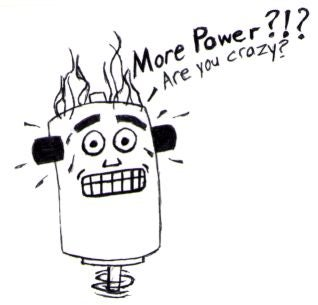There is normally no reason to operate a motor anywhere near this power point.

How accurate is our model?

Our newly completed simple motor model is reasonably accurate for most general cases.  It will fail to accurately predict some situations, such as when we are operating the motor at an extremely low RPM, at a current draw below the no-load current, or possibly near the motor's limitations.

The reason the model isn't perfect is because real motors do not have hard, fixed constants that govern their operation.  The armature resistance of a motor is modified by several factors during use.  Likewise, the no-load current is not a single current value.  It is dependent on input voltage, RPM, and other factors.   You will find in the real world that it is quite possible to run a motor below its no-load current rating, even though our simplified motor model predicts that the the shaft should not spin at all in this range.

However, we do not have to concern ourselves with the perfection of the model.   Our point is simply to provide a way to compare motors for the purpose of selecting the correct one, and to determine such things as the efficient operating range, which prop to use for which current, etc.  When we use our simplified motor model to compare values (and make reasonably accurate guesses) we are using it for the purpose it was designed for.

One caveat to the comparison concept is that brushless motors are treated a little unfairly by our model.  We do not take any kind of friction or second order heat effects into account.  This may make a brushed motor look identical to a brushless motor, but rest assured that a brushless motor has many advantages due to its lack of friction.  A brushless motor is much more likely to keep its armature resistance under control during operation and can be signficantly more efficient.

We are also not considering some critical non-performance factors, such as weight and price.   These are very important considerations when selecting a motor.  We will examine these things later and compare the motors based on performance alone at this point.

Summary

We have now completed our simple motor model which will allow us to predict motor performance.  The next step, detailed next month, is for us to learn how to predict power system performance using various real world propellers.  Its a little harder than you might expect.

This month we learned the following things:

• Power is a representation of how much work can be done in a given time.
• One horsepower is the same as 746 watts.
• Rotational power is expressed in terms of torque and RPM.  Torque is specified in inch-ounces and is a measure of how much "oomph" is behind the motor's shaft rotation.
• A motor's Kt value specifies its ability to produce torque for each input amp.  The torque constant, Kt, can be directly derived from the Kv of the motor.  It is impossible to increase the torque constant without proportionately decreasing the voltage constant of a motor (and vice-versa).
• A motor does not produce torque perfectly.  There is a torque loss equal to the no-load current value of the motor.  The no load current is symbolized using "Io".
• A motor is subject to two primary limitations: the RPM limit and the Torque limit.   There is no power limit, per se.
• Output power can be computed by taking the RPM and Torque losses into account.   Pout = (Vin - Iin * Rm) * (Iin - Io).
• Motor Efficiency is calculated by dividing Power Out by Power In.
• Increasing voltage always increases motor efficiency up until the point where the RPM limit is reached (when the efficiency drops to zero immediately!).
• Increasing current increases the RPM losses and decreases the torque losses.
• The point at which the RPM loss equals the torque loss is the maximum efficiency point of the motor.
• Our simplified motor model is best used for comparison of different motors and for making reasonably accurate guesses.

Q&A

Joe's Super Thermal 2000 R/C electric sailplane now has a new motor that will let it climb out vertically to 500 feet.  Joe's sailplane weighs 5 lbs.  How much work is accomplished during the vertical climb?  How much power is the motor producing if the climb takes 10 seconds?

Suppose I have a motor with a Kv of 1500.  What is the Kt value?

Consider the following motors:

Motor1: Kv = 4000, Io = .2 amps, Rm = .150 Ohms
Motor2: Kv = 2000, Io = 2 amps, Rm = .050 Ohms

Which motor is best for an indoor flyer that will draw only 2 amps on 6 cells?   What will be the power out and efficiency of the chosen motor?

Which motor is best for a sport plane that will draw 30 amps on 10 cells?  What will be the power out and efficiency of the chosen motor?

Suppose I have a motor with an RPM limit of 30,000.  With a certain battery pack and a 50 amp current draw, this motor is able to spin a 12x8 propeller at 29,000 RPM.   If I remove the propeller and rev up the motor, what will happen?

Look on the internet at the following websites: www.maxcim.com,   www.aveox.com, www.astroflight.com.  All three of these websites list motors with their constants.  Compare the constants, price, and weight of the various motors.  Do you see any trends?

 Thread Tools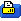Show Printable Version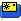Email this Page